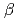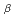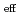# Effect ofon effective multiplication factor in 1/fspectrum random system
We investigated thedependence of the effective multiplication factor (k) in the 1/fnoise model. We conducted the two-group Monte Carlo calculations. We found that the standard deviation of the kdistribution showed the positive correlation with thevalue because the spatial distribution of the fuel became less uniform as thevalue increased.